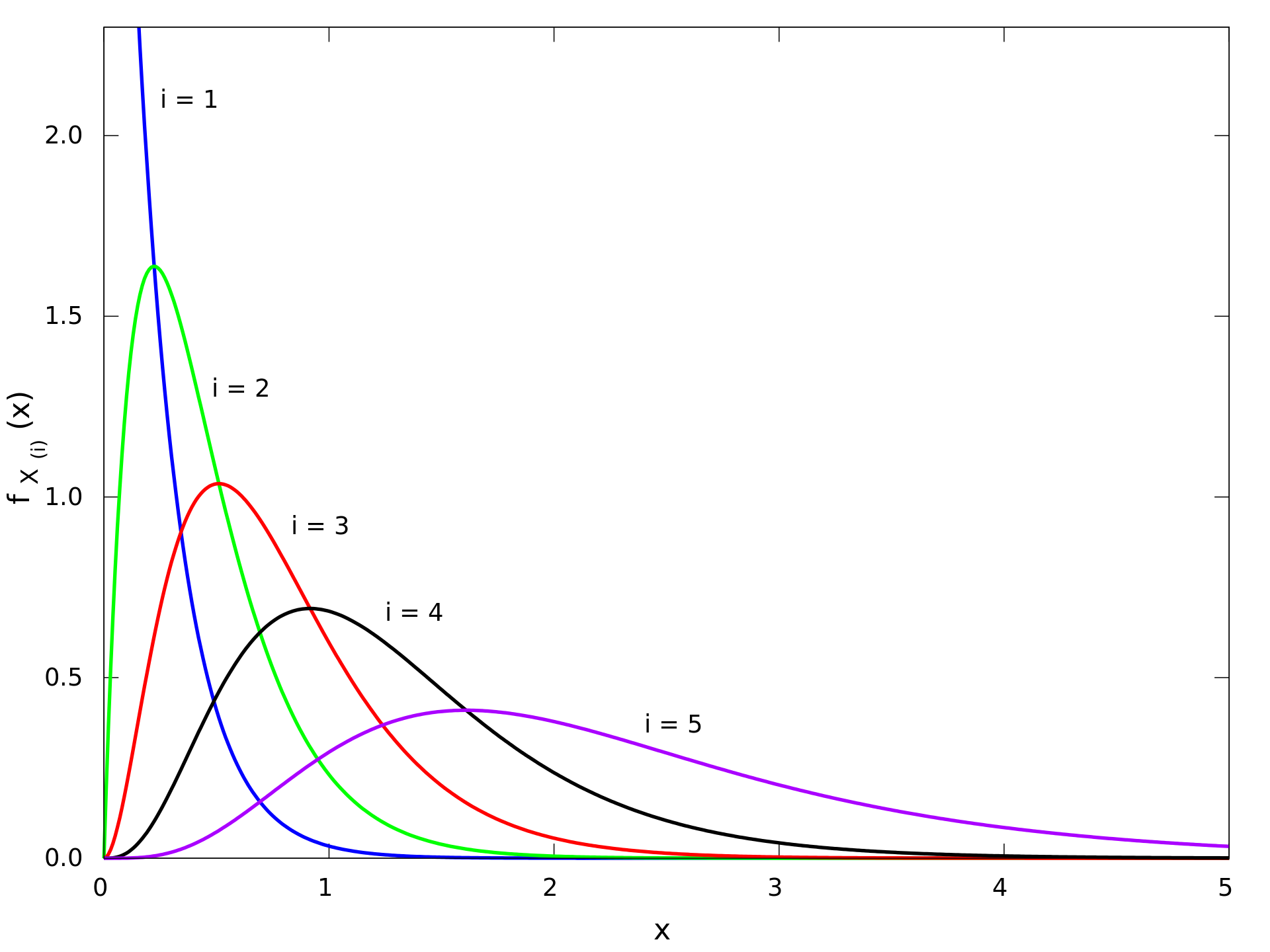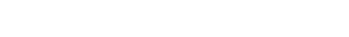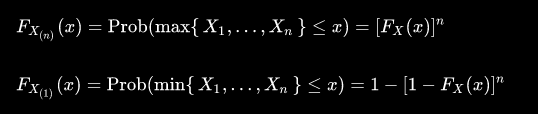## 10_R

### Distributions of the order statistics## 10_R assignament

### Request

Distributions of the order statistics: look on the web for the most simple (but still rigorous) and clear derivations of the distributions, explaining in your own words the methods used.

### Order statistics

Together with rank statistics, order statistics are among the most fundamental tools in non-parametric statistics and inference. In statistics we define the kth order statistic of a statistical sample as its kth-smallest value.

Important special cases of the order statistics are the minimum and maximum value of a sample, and the sample median and other sample quantiles.

When using probability theory to analyze order statistics of random samples from a continuous distribution, the cumulative distribution function is used to reduce the analysis to the case of order statistics of the uniform distribution.Probability density functions of the order statistics for a sample of size n = 5 from an exponential distribution with unit scale parameter

### Distributions function of order statistic

For a random sample as above, with cumulative distribution Fx(x), the order statistics for that sample have cumulative distributions as follows (where r specifies which order statistic):the corresponding probability density function may be derived from this result, and is found to be:Moreover, there are two special cases, which have CDFs which are easy to compute.Which can be derived by careful consideration of probabilities.